﻿ 手术微器械盲孔特征的腹腔镜视野自动调节方法
«上一篇文章快速检索 高级检索

 哈尔滨工程大学学报2019, Vol. 40Issue (8): 1495-1501  DOI: 10.11990/jheu.2018071200

### 引用本文YU Lingtao, ZHENG Hongjie. Automatic adjustment of the laparoscopic visual field for blind-hole-like surgical instruments[J]. Journal of Harbin Engineering University, 2019, 40(8), 1495-1501. DOI: 10.11990/jheu.201807120.### 文章历史

1. 哈尔滨工程大学 机电工程学院, 黑龙江 哈尔滨 150001;
2. 上海空间电源研究所, 上海 201100

Automatic adjustment of the laparoscopic visual field for blind-hole-like surgical instruments
YU Lingtao 1, ZHENG Hongjie 21. College of Mechanical and Electrical Engineering, Harbin Engineering University, Harbin 150001, China;
2. Shanghai Institute of Space Power-Sources, Shanghai 201100, China
Abstract: This work proposes a method for the automatic adjustment of the laparoscopic visual field based on blind-hole-like surgical instruments. The proposed method can provide the optimal surgical field of vision and will improve the efficiency of robot-assisted minimally invasive surgery by improving the autonomy of operation tasks and relieving the pressure on the surgeon during surgery. First, the coordinate systems of the laparoscopic imaging system and the camera and the laparoscopic motion-imaging relationship are derived through the D-H method in accordance with the coordinate system of the endoscope arm. Then, the characteristics of blind-hole-like surgical instruments are captured by using the machine vision algorithm to obtain the size and position in the image coordinate system. Finally, the laparoscope visual field is automatically adjusted by combining relative size and surgical instrument position. Experiments are performed with an abdominal surgery robot prototype. Experimental results prove that the method can enable the automatic adjustment of the laparoscopic field of view and verify the correctness and superiority of the proposed method.
Keywords: surgical robot    machine vision    robot kinematics    object tracking    laparoscopic vision    automatic adjustment    experimental analysis

1 问题描述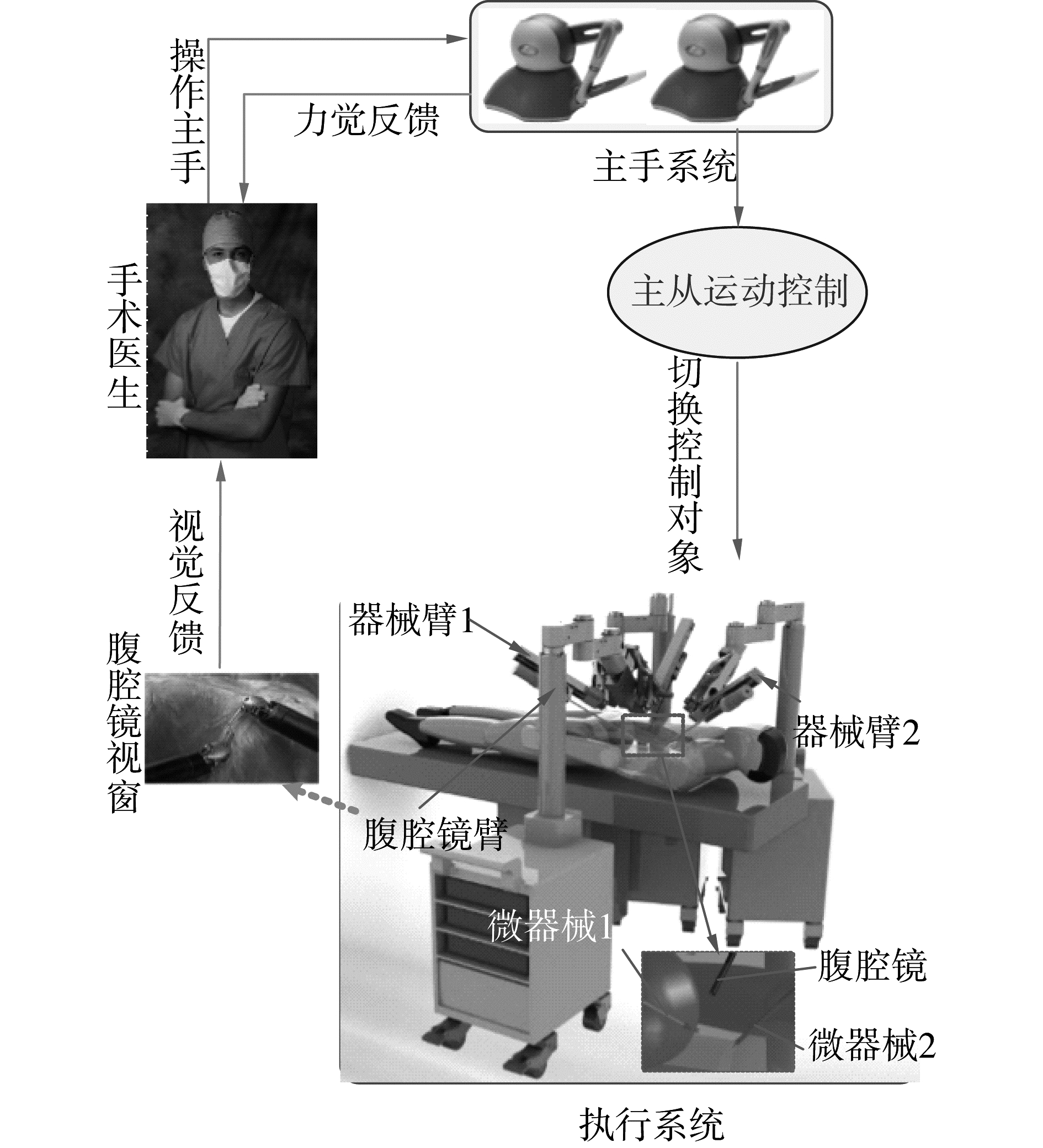Download: 图 1 微创外科手术机器人系统基本结构组成 Fig. 1 Basic structure of minimally invasive surgical robot system

2 手术微器械检测方法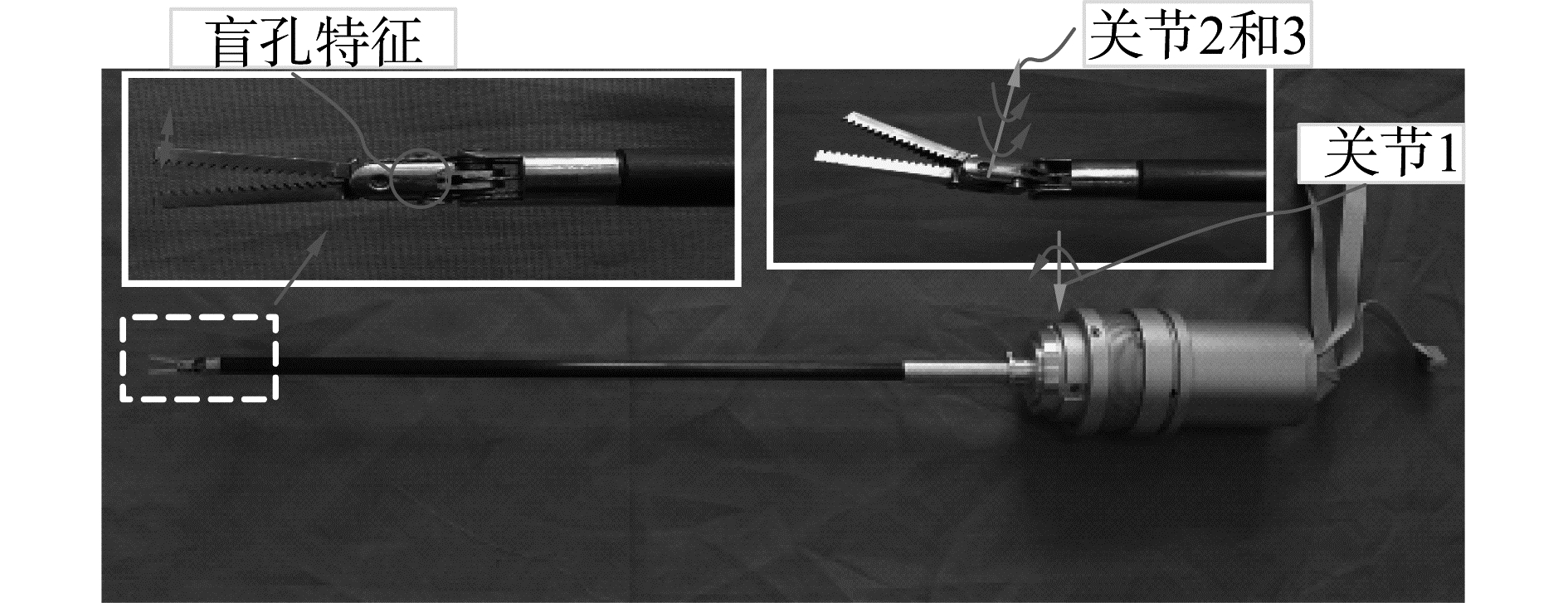Download: 图 2 3自由度手术微器械及其盲孔特征 Fig. 2 The 3-DOF micro surgical instrument and its blind hole feature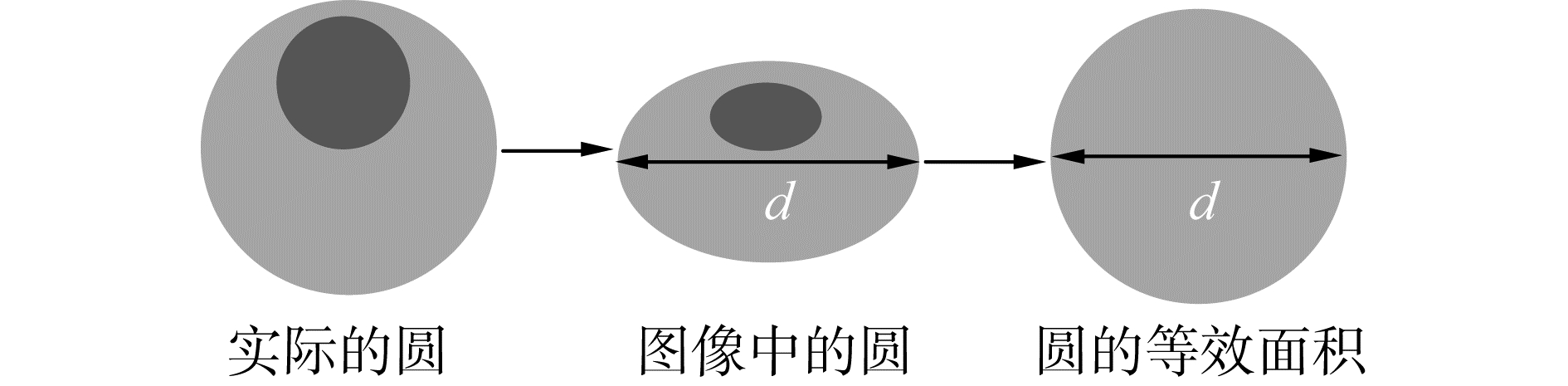Download: 图 3 圆特征的等效过程 Fig. 3 Equivalent process of circle characteristics
2.1 手术微器械类似盲孔特征检测Download: 图 4 手术微器械类似盲孔特征简化结构 Fig. 4 Simplified structure of micro surgical instrument's blind hole feature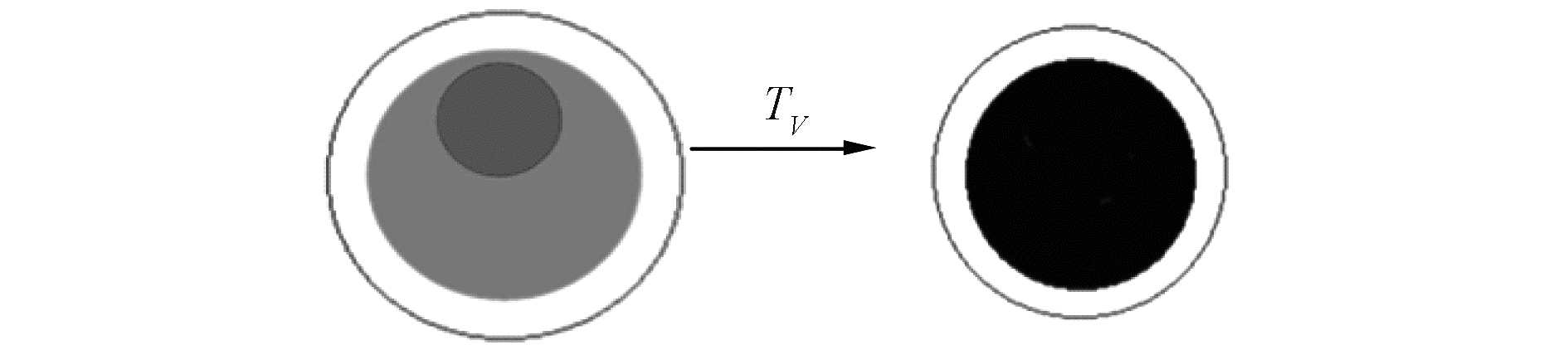Download: 图 5 手术微器械类似盲孔特征图像分割过程 Fig. 5 Image segmentation of micro instrument's blind hole feature

 $p\left( {i,j} \right) = \left\{ \begin{array}{l} 0.9,\;\;\;P\left( {i,j} \right) \le g\\ 0,\;\;\;\;\;\;P\left( {i,j} \right) > g \end{array} \right.$ (1)

 $\begin{array}{*{20}{c}} {{b^2}{{\left( {\left( {i - i'} \right)\cos \theta + \left( {j - j'} \right)\sin \theta } \right)}^2} + }\\ {{a^2}{{\left( { - \left( {i - i'} \right)\sin \theta + \left( {j - j'} \right)\cos \theta } \right)}^2} - {a^2}{b^2} = 0} \end{array}$ (2)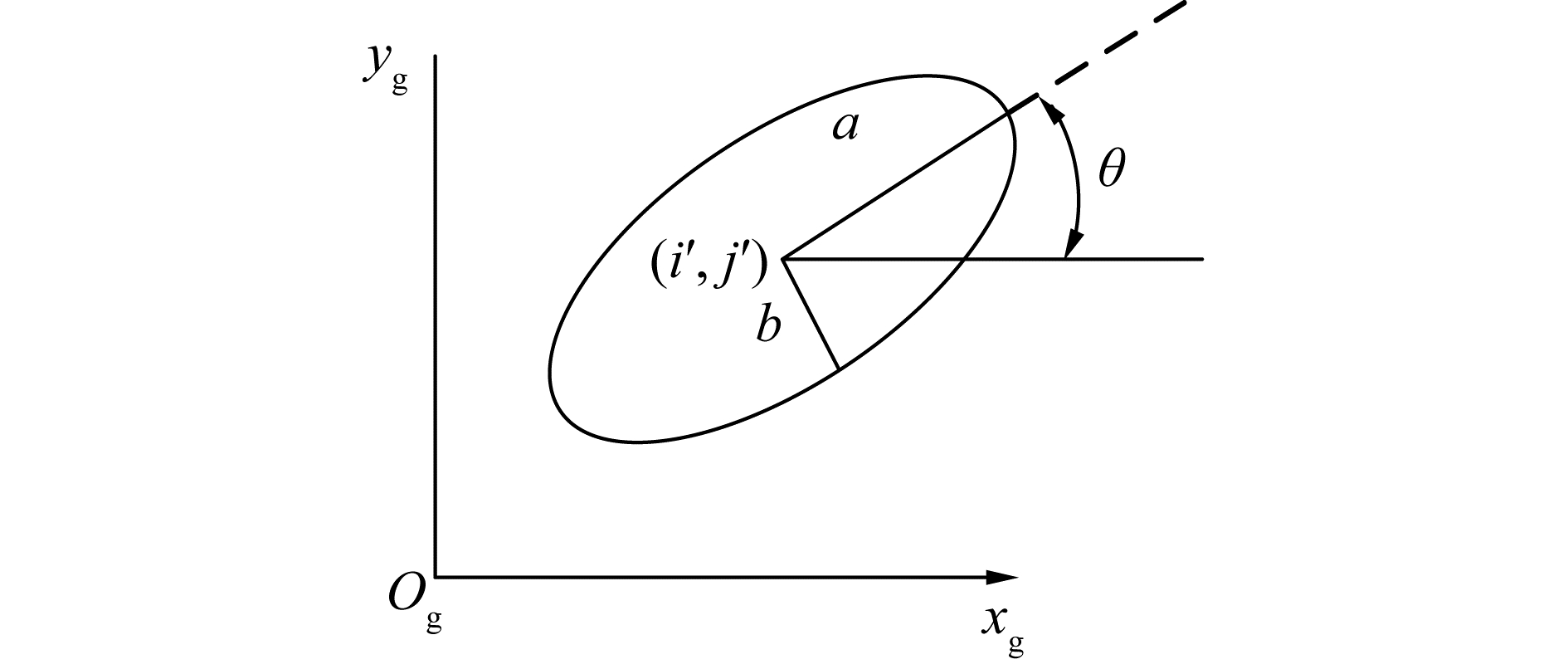Download: 图 6 图像坐标系下椭圆的示意 Fig. 6 Schematic diagram of an ellipse in an image coordinate system

 $\left\{ \begin{array}{l} h = \sqrt {\frac{{{a^2}{{\tan }^2}\theta + {b^2}}}{{{{\tan }^2}\theta + 1}}} \\ w = \sqrt {\frac{{{b^2}{{\tan }^2}\theta + {a^2}}}{{{{\tan }^2}\theta + 1}}} \end{array} \right.$ (3)
2.2 改进的机器视觉算法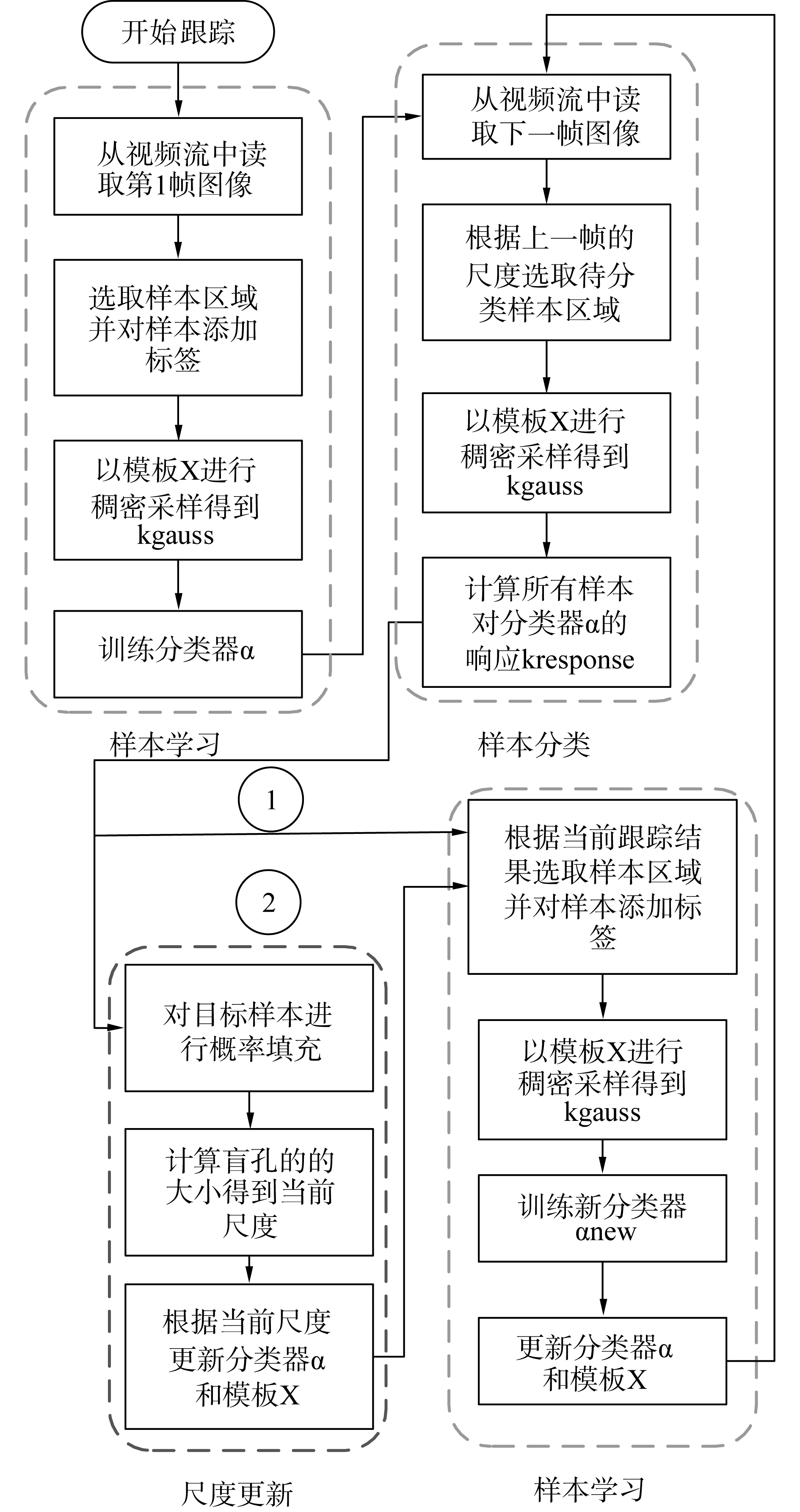Download: 图 7 CSK目标跟踪算法及其改进算法流程 Fig. 7 The flow chart of CSK target tracking algorithm and its improved algorithm

 ${w_{\rm{n}}} = pw;{h_{\rm{n}}} = ph$ (4)

3 持镜臂系统运动成像关系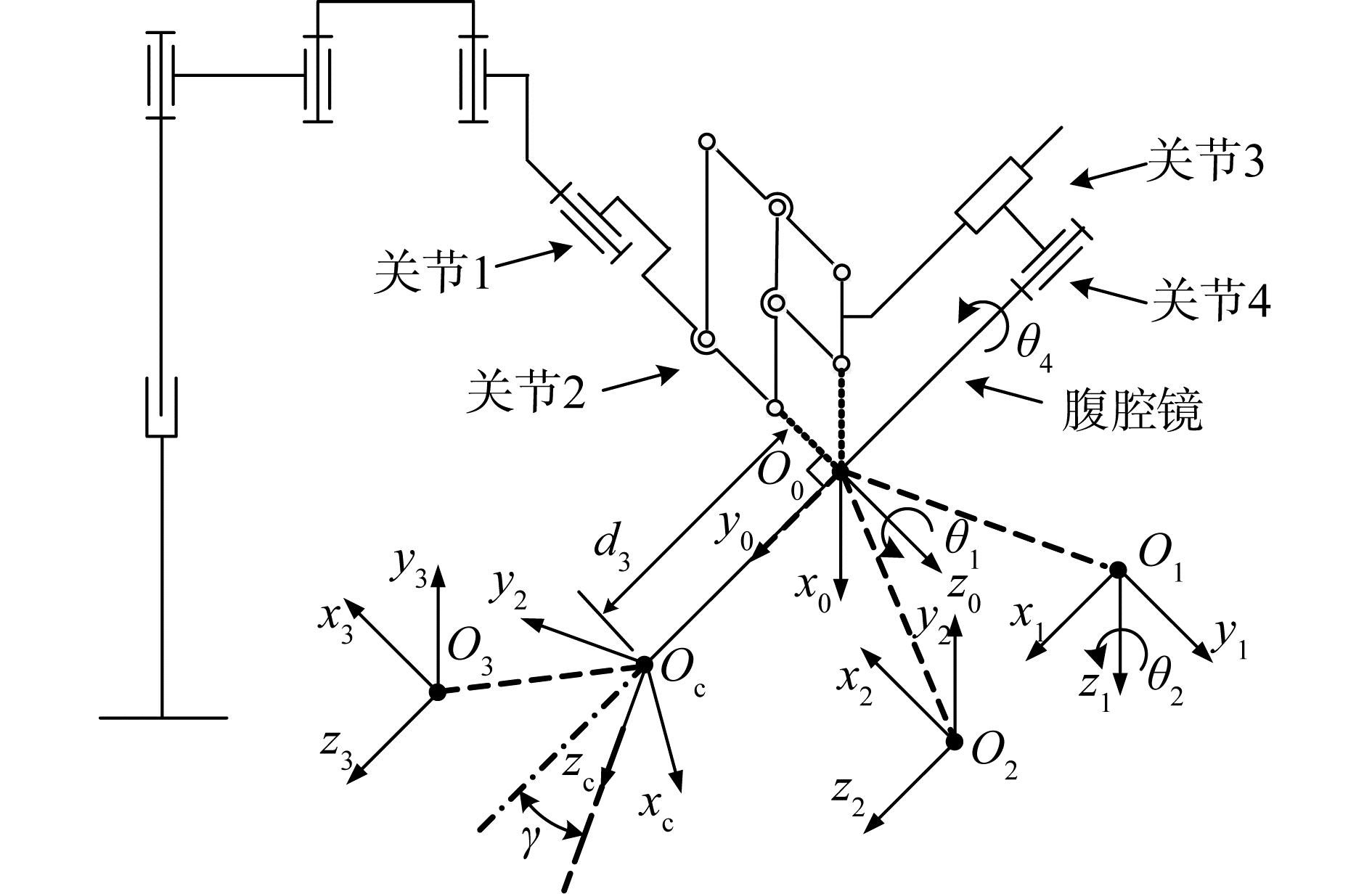Download: 图 8 持镜臂结构示意及其运动学坐标系 Fig. 8 The structure sketch map and the kinematic coordinate system of mirror arm表 1 运动学坐标系的连杆参数 Table 1 Linkage parameters of kinematic coordinate system

 $\begin{array}{*{20}{c}} {{}^{i - 1}{\mathit{\boldsymbol{T}}_i} = {T_{z,d}}{R_{z,\theta }}{T_{x,a}}{R_{x,\alpha }} = }\\ {\left[ {\begin{array}{*{20}{c}} {\cos {\theta _i}}&{ - \cos {\alpha _i}\sin {\theta _i}}&{\sin {\alpha _i}\sin {\theta _i}}&{{a_i}\cos {\theta _i}}\\ {\sin {\theta _i}}&{\cos {\alpha _i}\cos {\theta _i}}&{ - \sin {\alpha _i}\cos {\theta _i}}&{{a_i}\cos {\theta _i}}\\ 0&{\sin {\alpha _i}}&{\cos {\alpha _i}}&{{d_i}}\\ 0&0&0&1 \end{array}} \right]} \end{array}$ (5)

 ${}^0{\mathit{\boldsymbol{T}}_{\rm{C}}}\left( {{\theta _1},{\theta _2},{d_3}} \right) = {}^0{T_1}{}^1{T_2}{}^2{T_3}{}^3{T_{\rm{C}}} =\\ \left[ {\begin{array}{*{20}{c}} {\cos {\theta _1}}&{ - \sin \left( {{\theta _2} + \gamma } \right)\sin {\theta _1}}&{ - \cos \left( {{\theta _2} + \gamma } \right)\sin {\theta _1}}&{ - {d_3}\cos {\theta _2}\sin {\theta _1}}\\ {\sin {\theta _1}}&{\sin \left( {{\theta _2} + \gamma } \right)\cos {\theta _1}}&{\cos \left( {{\theta _2} + \gamma } \right)\cos {\theta _1}}&{{d_3}\cos {\theta _2}\cos {\theta _1}}\\ 0&{ - \cos \left( {{\theta _2} + \gamma } \right)}&{\sin \left( {{\theta _2} + \gamma } \right)}&{{d_3}\sin {\theta _2}}\\ 0&0&0&1 \end{array}} \right]$ (6)

 ${}^g{\mathit{\boldsymbol{P}}_{{\rm{mn}}}} = \left[ {\begin{array}{*{20}{c}} {{x_{{\rm{mn}}}}}\\ {{y_{{\rm{mn}}}}} \end{array}} \right] = \left[ {\begin{array}{*{20}{c}} {\left( {{x_{{\rm{Mn}}}}/{z_{{\rm{Mn}}}}} \right){f_x} + {c_x}}\\ {\left( {{y_{{\rm{Mn}}}}/{z_{{\rm{Mn}}}}} \right){f_y} + {c_y}} \end{array}} \right]$ (7)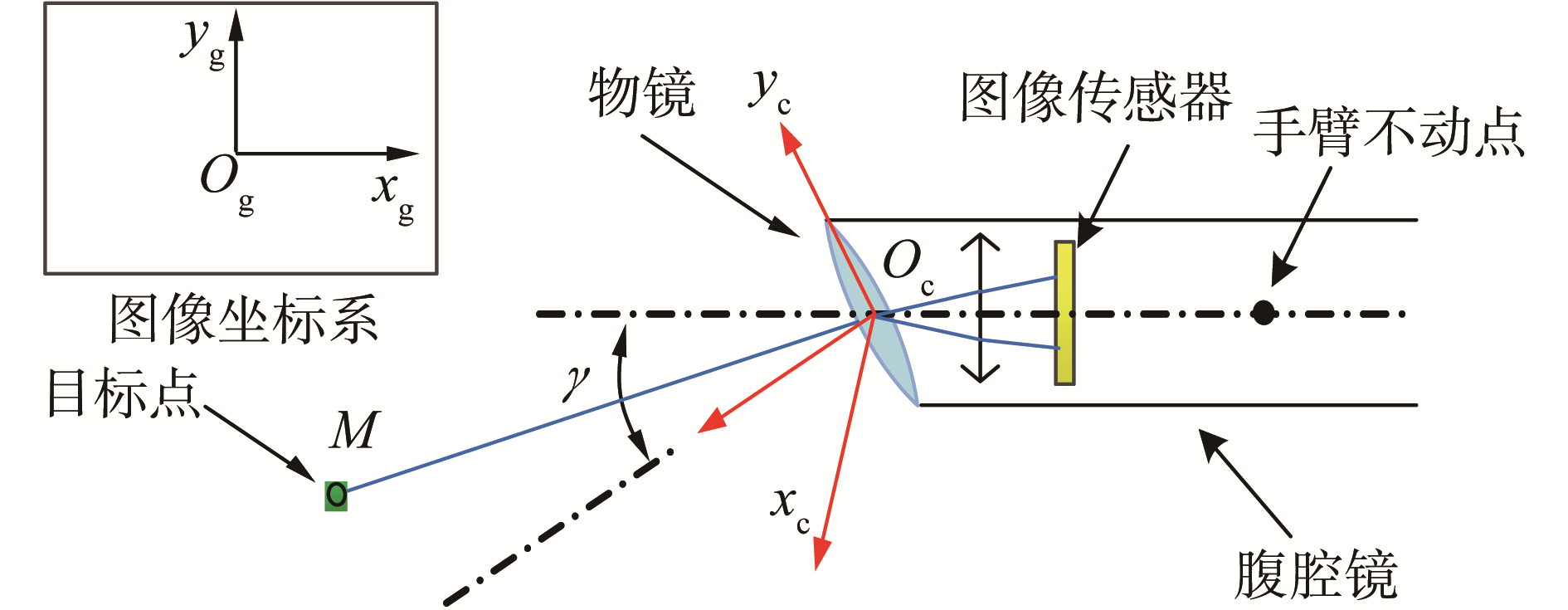Download: 图 9 腹腔镜的成像简化模型 Fig. 9 Simplified model of laparoscopic imaging

 $\left\{ \begin{array}{l} \left( {{x_{{\rm{Mn}}}}/{z_{{\rm{Mn}}}}} \right){f_x} = 0\\ \left( {{y_{{\rm{Mn}}}}/{z_{Mn}}} \right){f_y} = 0 \end{array} \right.$ (8)

 $\left\{ \begin{array}{l} {x_{Mn}} = {f_x}\left( \alpha \right)\\ {y_{Mn}} = {f_y}\left( {\alpha ,\beta ,\Delta d} \right)\\ {z_{Mn}} = {f_z}\left( {\alpha ,\beta ,\Delta d} \right) \end{array} \right.$ (9)

4 腹腔镜视野自动调节规则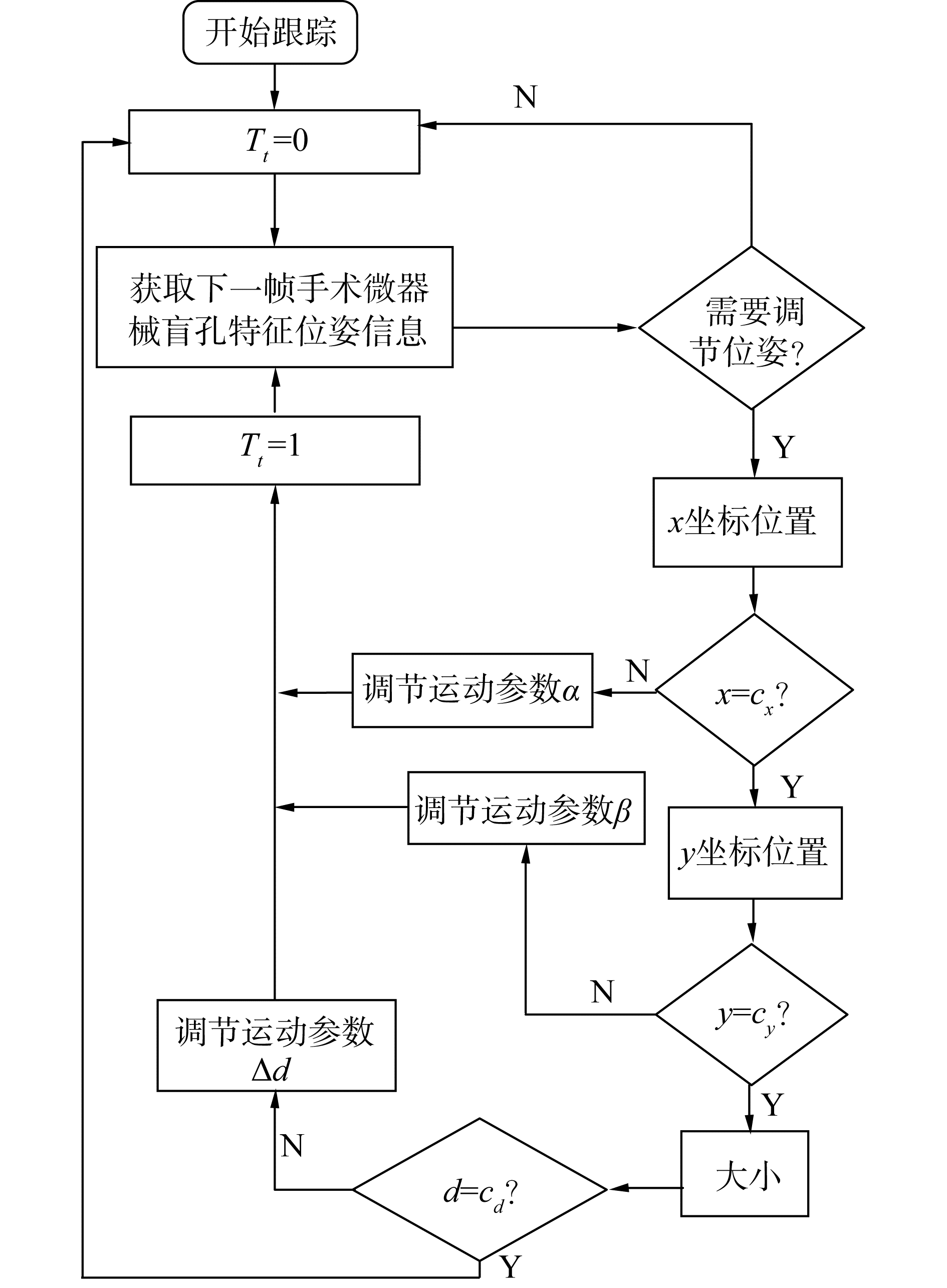Download: 图 10 腹腔镜视野自动调节规则 Fig. 10 Automatic adjustment rule of laparoscopic visual field

 $\left\{ \begin{array}{l} \left( {{x_L} < = {T_{\rm{w}}}\left\| {{x_R}} \right. > = \left( {{\rm{width}} - {T_{\rm{w}}}} \right)\left\| {{y_T} < = } \right.\left. {{T_h}} \right\|} \right.\\ {y_D} > = \left( {{\rm{height}} - {T_{\rm{h}}}} \right)\left\| {d < = {T_{{\rm{dll}}}}\left\| {d > = {T_{{\rm{dh}}}}} \right\|} \right.\\ \left. {{T_t} = = 1} \right) \end{array} \right.$ (10)Download: 图 13 腹腔镜成像中腹腔镜视野自动调节规则参数 Fig. 13 Automatic adjustment rule' parameters of laparoscopic visual field in laparoscopic imaging

 $\left\{ \begin{array}{l} {x_{\rm{L}}} = {\rm{MIN}}\left( {{x_1} - {d_1},{x_2} - {d_2}} \right)\\ {x_{\rm{R}}} = {\rm{MAX}}\left( {{x_1} + {d_1},{x_2} + {d_2}} \right)\\ {y_{\rm{T}}} = {\rm{MIN}}\left( {{y_1} - {d_1},{y_2} - {d_2}} \right)\\ {y_{\rm{D}}} = {\rm{MAX}}\left( {{y_1} + {d_1},{y_2} + {d_2}} \right) \end{array} \right.$

 $\left\{ \begin{array}{l} {c_x} \in \left( {{\rm{width}}/2 - {T_c},{\rm{width}}/2 + {T_c}} \right)\\ {c_y} \in \left( {{\rm{height}}/2 - {T_c},{\rm{height}}/2 + {T_c}} \right)\\ {c_d} \in \left( {{T_{{\rm{dl}}}},{T_{{\rm{dh}}}}} \right) \end{array} \right.$ (11)
5 实物实验及实验分析Download: 图 11 腹腔镜视野自动调节模拟实验系统 Fig. 11 Simulation experiment system of laparoscopic visual field′s automatic adjustment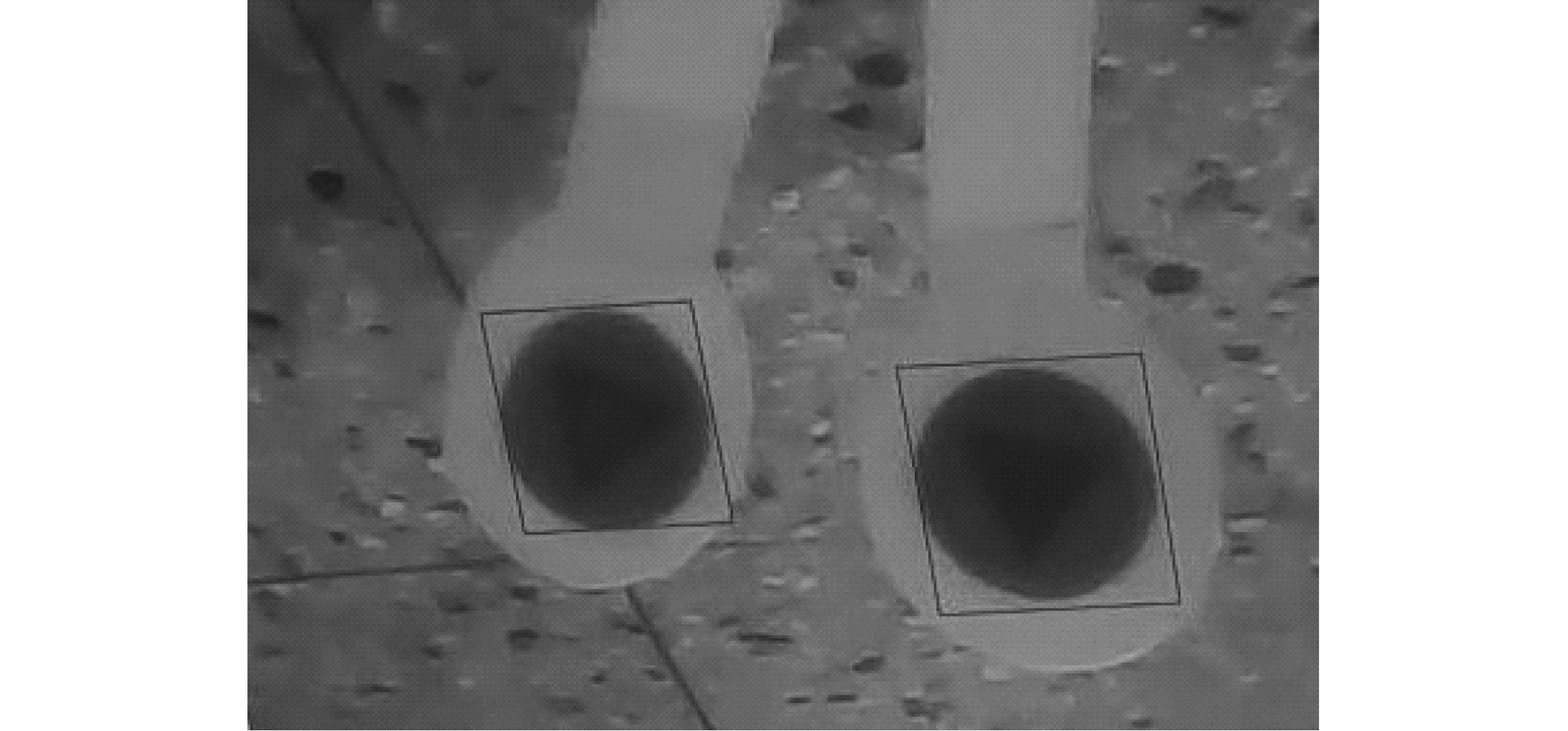Download: 图 12 选定初始目标区域 Fig. 12 Selection initial target areaDownload: 图 14 手术微器械在腹腔镜成像坐标中x方向即将超出视野时自动调节 Fig. 14 Automatic adjustment of laparoscopic visual field when the surgical instruments are about to exceed its vision in the direction of x in the coordinate of laparoscopic imagingDownload: 图 15 手术微器械在腹腔镜成像坐标中y方向即将超出视野时自动调节 Fig. 15 Automatic adjustment of laparoscopic visual field when the surgical instruments are about to exceed its vision in the direction of y in the coordinate of laparoscopic imagingDownload: 图 16 手术微器械在腹腔镜成像坐标中大小不合适时自动调节 Fig. 16 Automatic adjustment of laparoscopic visual field when the size of surgical instruments is not appropriate

6 结论

1) 面向具有持镜臂和持械臂的腹腔镜微创手术机器人系统，本文详细阐述了实现腹腔镜视野调节自动化核心问题的解决方法，使腹腔镜手术机器人能够为医生自主提供最佳的手术视野。基于手术微器械自身颜色，结合改进的CSK跟踪算法，对类似盲孔特征进行跟踪检测，提高了手术微器械位姿检测的精准性和稳定性；

2) 采用D-H法对持镜臂系统进行运动学建模，结合腹腔镜成像原理，分析运动成像关系，从而制定腹腔镜视野自动调节规则；结合上述手术微器械的位姿参数和腹腔镜视野自动调节规则，通过实物实验验证了本方法的正确性和可行性；

3) 本文提出的腹腔镜视野自动调节方法具有一定的理论意义与实用价值，不受机械传动误差的影响，可以提高腹腔镜微创手术机器人系统在进行微创手术时的自主性、灵活性和可靠性，它不仅可以作为腹腔镜微创手术机器人系统腹腔镜视野调节的专用方法，还可以用于其他具有视觉反馈的主从控制机器人系统。

  BALLESTER P, JAIN Y, HAYLETT K R, et al. Comparison of task performance of robotic camera holders EndoAssist and Aesop[J]. International congress series, 2001, 1230: 1100-1103. DOI:10.1016/S0531-5131(01)00231-X (0)  徐兆红, 宋成利, 闫士举. 机器人在微创外科手术中的应用[J]. 中国组织工程研究与临床康复, 2011, 15(35): 6598-6601. XU Zhaohong, SONG Chengli, YAN Shiju. Research on minimally invasive robotic surgery[J]. Journal of Clinical rehabilitative tissue engineering research, 2011, 15(35): 6598-6601. DOI:10.3969/j.issn.1673-8225.2011.35.033 (0)  BRAHMBHATT J V, GUDELOGLU A, LIVERNEAUX P, et al. Robotic microsurgery optimization[J]. Archives of Plastic Surgery, 2014, 41(3): 225-230. DOI:10.5999/aps.2014.41.3.225 (0)  LATIF H O, SHERKAT N, LOTF A. TeleGaze: teleoperation through eye gaze[C]//Proceedings of the 2008 7th IEEE International Conference on Cybernetic Intelligent Systems. London, UK, 2008: 1-6. (0)  MUDUNURI A V. Autonomous camera control system for surgical robots[D]. Detroit, USA: Wayne State University, 2010. (0)  AZIZIAN M, KHOSHNAM M, NAJMAEI N, et al. Visual servoing in medical robotics:a survey. Part Ⅰ:endoscopic and direct vision imaging-techniques and applications[J]. International Journal of medical robotics and computer assisted surgery, 2014, 10(3): 263-274. DOI:10.1002/rcs.v10.3 (0)  KING B W, REISNER L A, PANDYA A K, et al. Towards an autonomous robot for camera control during laparoscopic surgery[J]. Journal of laparoendoscopic & advanced surgical techniques, 2013, 23(12): 1027-1030. (0)  WEEDE O, MÖNNICH H, MVLLER B, et al. An intelligent and autonomous endoscopic guidance system for minimally invasive surgery[C]//Proceedings of 2011 IEEE International Conference on Robotics and Automation. Shanghai, 2011: 5762-5768. (0)  丁业兵. 基于惯量矩的椭圆拟合方法[J]. 计算机工程与应用, 2016, 52(18): 188-191. DING Yebing. Ellipse fitting method based on moment of inertia[J]. Computer engineering and applications, 2016, 52(18): 188-191. DOI:10.3778/j.issn.1002-8331.1412-0056 (0)  NIXON M S, AGUADO A S.特征提取与图像处理[M].李实英, 杨高波, 译. 2版.北京: 电子工业出版社, 2010: 255-258. NIXON M S, AGUADO A S. Feature extraction and image processing[M]. LI Shiying, YANG Gaobo, trams. 2nd ed. Beijing: Electronic Industry Press, 2010: 255-258. (0)  HENRIQUES J F, CASEIRO R, MARTINS P, et al. Exploiting the circulant structure of tracking-by-detection with kernels[C]//Proceedings of the 12th European Conference on Computer Vision. Florence, Italy, 2012: 702-715. (0)  钱元凯. 摄影光学与镜头[M]. 杭州: 浙江摄影出版社, 2005. QIAN Yuankai. The photographic optics & lenses[M]. Hangzhou: Zhejiang Photography Publishing House, 2005. (0)  BRADSKI G, KAEHLER A.学习OpenCV(中文版)[M].于仕琪, 刘瑞祯, 译.北京: 清华大学出版社, 2009: 407-409. BRADSKI G, KAEHLER A. Learning OpenCV[M]. YU Shiqi, LIU Ruizhen, trans. Beijing: Tsinghua University Press, 2009: 407-409. (0)